# Mixture of nuts

Alice bought a mixture of nuts containing cashew nuts, hazelnuts and peanuts in a ratio of 1: 2: 3. Calculate the weight in grams of the whole mixture if the peanuts weigh 90 g.

x =  180 g

### Step-by-step explanation: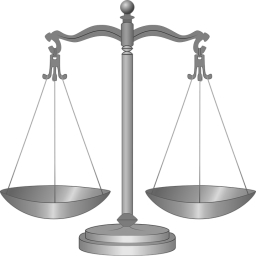Did you find an error or inaccuracy? Feel free to write us. Thank you!Tips to related online calculators
Check out our ratio calculator.

## Related math problems and questions:

• Mixture of nutsThe mixture of nuts should be prepared from almonds, peanuts and cashew nuts ratio 1: 2: 3 (respectively). The price of almonds is 150 CZK/kg, the price of peanuts is 140 CZK/kg and the price of cashew nuts is 180 CZK/kg. The price of the mixture is deter
• Tea blendTea blends are maked from two kinds of tea. In standard tea mixture are two teas in the ratio 1:3 and 40 g costs 42 CZK. In the premium tea mixture are weighing two teas in the ratio 1:1 and 50 grams costs 60 CZK. How much cost 10 grams of more expensive
• A 36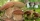A 36 g sample of the substance contains 91% water. After drying, the weight of the sample was reduced to 18 g. What percentage of water is now in the sample?
• Acid solutionBy adding 250 grams of a 96% sulfuric acid solution to its 3% solution, we changed its initial concentration to 25%. How many grams of 3% of the acid was used for dilution?
• Three friends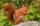Three friends squirrels together went to collect hazelnuts. Zrzecka he found more than twice Pizizubka and Ouska even three times more than Pizizubka. On the way home they talked while eating and was cracking her nuts. Pizizubka eaten half of all nuts whi
• Shen'sShen's chocolate bar is 54% cocoa. If the weight of the chocolate bar is 54 grams, how many grams of cocoa does it contain? Round your answer to the nearest tenth.
• Alloy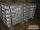The first alloy is a mixture of two metals in the ratio 1:2, the second is a mixture of same metals in the ratio 2:3. At what ratio we have these two alloys put into the furnace to obtain a new metal alloy with ratio 17:27? (All three ratios correspond to
• Nutballs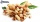The dough for nutballs contains, among other things, two basic raw materials: flour and nuts in a ratio of 2:1. How much is flour, and how many nuts are needed for 1 kg of dough if "other" is 100g?
• CuSO4 mixtureHow many grams of solid CuSO4 we have to add to 450g of 15% CuSO4 solution to produce a 25% solution?
• R1+R2 in parallelThe resistance ratio of the first and second resistors is 3: 1 and the current flowing through the first resistor is 300 mA. What current flows through the second resistor (resistors connected in parallel)?
• CoffeeIn-stock are three kinds of branded coffee prices: I. Kind. .. .. .205 Kc/kg II. Kind. .. .. .274 Kc/kg III. Kind. .. .. 168 Kc/kg Mixing these three species in the ratio 8:5:6 create a mixture. What will be the price of 100 grams of this mixture?
• Alcohol mixtureThree liters of 96 percent alcohol set up a certain amount of distilled water to form 54-percent alcohol. How many liters of distilled water was used?
• MushroomsMushrooms lose 90% by weight drying. How many fresh mushrooms are needed for 5 kg of dried mushrooms?
• NutsPeter has 49 nuts. Walnuts have 3 more than hazelnuts and hazelnut 2 over almonds. Determine the number of wallnuts, almonds and hazelnuts.
• GramsHow many grams of 5% solution do we need to add to 100g of 50% solution to get a 20% solution?
• Alcohol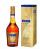How many cubic centimeters of 96% alcohol is needed to obtain 0.75 liters of 40% alcohol?
• MixtureHow many percentages mixtures do we get by adding 23ml of matter to 89ml of water?